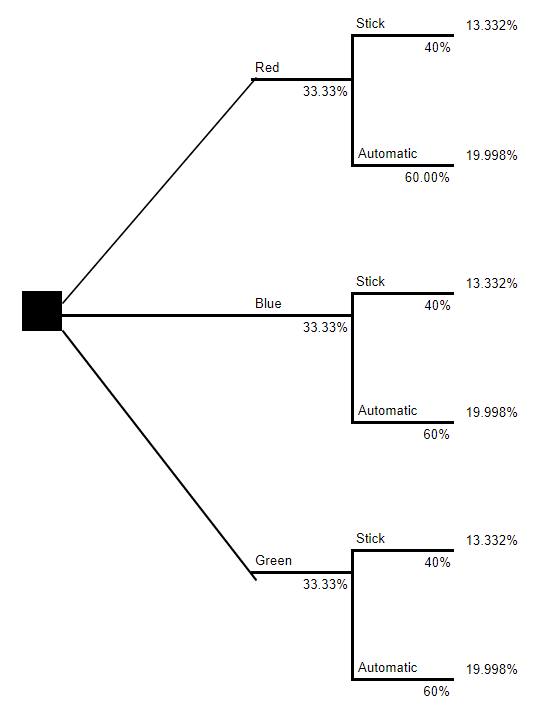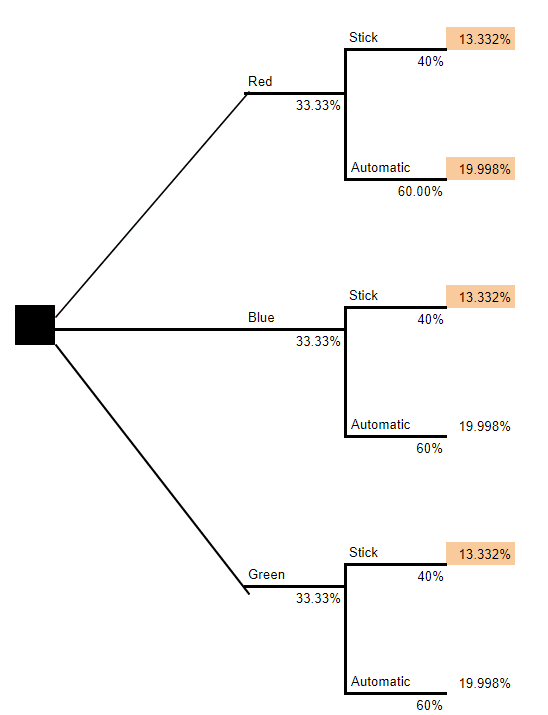# Probabilities and Distinctions

Manipulating probabilities mathematically is very simple. If the question you’re asking is an “and” question, you multiply. If the question you’re asking is an “or” question, you add.

Let’s go through an example.

• A parking lot is full of an equal number of red, green, and blue vehicles
• In your current location, 40% of vehicles are stick shifts (manual transmission)

What are the odds that any randomly selected vehicle will be blue and a stick shift?

This is an “and” question, so you multiply 0.3333 x 0.40 = 0.1333 or 13.33%.Now, what are the odds that a particular randomly sampled vehicle is an automatic transmission, or is red? This is not actually so straightforward. I can’t calculate the right answer in my head and this is a relatively simple question.The best way to approach a complex problem is to quickly build a tree. In a probability tree, each “branch” corresponds to a distinction. A distinction is a meaningful difference that you need to keep track of. The first distinction is the color. The second distinction is the transmission type, stick v.s automatic. You can easily calculate the odds of each terminal node (the “leaves”) of the probability tree by multiplying down the branch back to the origin.

We already implicitly did this when we asked about the odds of a random sample being blue and a stick shift. We only needed to think about one “leaf”, going from the origin, through “blue” and “stick shift”, so it was simple.

Returning to the question: What are the odds that a particular randomly sampled vehicle is an automatic transmission, or is red?

The way to answer this is to add up the terminal nodes corresponding to the categories you’re looking for. And, interestingly, we’ve now uncovered an annoying ambiguity in English. Because it’s not clear whether the node corresponding to both “Red” and “Stick” qualifies. Did we mean or-inclusive, or did we mean or-exclusive? If we meant or-inclusive, then a red-stickshift car qualifies as “red or stickshift”, but if we meant or-exclusive, then it doesn’t! We need to learn to be sharp about the difference between the two senses of “or.” Let’s say that for the purposes of our question and what we’re interested in finding out, we mean or-inclusive.That means we selected the nodes that satisfy our criteria (stickshift, red, or both) and add these numbers (highlighted) together. The sum is 60%.

## Join the Guild

Seedling
$0/month Structure your self-improvement. 15 Path levels per Archetype Unlimited workshops per month Full community access One cohort* Flower$15/month